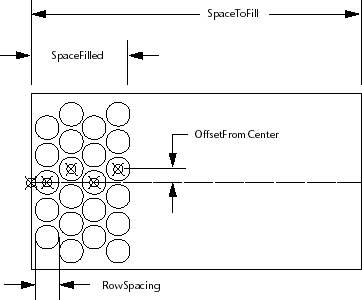# Applying Some Logic

Applying Some Logic

One thing you need to do is determine how to space out the tiles and draw them. If this were a simple rectilinear grid of tiles, you could use the AutoCAD® ARRAY command to fill in the tiles. But for the garden path, you need to have each row of tiles offset from the previous row.

This row-offset pattern is a repeating pattern. Think of how you might go about laying the tiles if you were building the actual path. You would probably be inclined to start at one end and just keep laying down rows until there wasn't any more space left.Here is the logic in pseudo-code:

`At the starting point of the path`
`Figure out the initial row offset from center (either centered on`
`the path or offset by one "tile space").`
`While the space of the boundary filled is less than the space to`
`fill,`
`  Draw a row of tiles.`
`  Reset the next start point (incremented by one "tile space").`
`  Add the distance filled by the new row to the amount of space`
`  filled.`
`  Toggle the offset (if it is centered, set it up off-center, or`
`  vice versa).`
`  Go back to the start of the loop.`